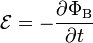Lenz Law - Electrical Engineering Gate

# Electrical Engineering Gate

Online Electrical Engineering Study Site

## Lenz's Law

In 1835 Heinrich Lenz stated the law that now bears his name.  An electric current induced by a changing magnetic field will flow such that it will create its own magnetic field that opposes the magnetic field that created it.  These opposing fields occupying the same space at the same time result in a pair of forces.   These forces are felt when you turn a generator and generate electricity.  The more current you generate, the greater the force opposing you
Lenz's law states that when an emf is generated by a change inmagnetic flux according to Faraday's Law, the polarity of the induced emf is such, that it produces an current that's magnetic field opposes the change which produces it
Lenz's law is shown with the negative sign in Faraday's law of induction$\mathcal{E}=-\frac{\partial \Phi_\mathrm{B}}{\partial t}$
which indicates that the induced emf (ℰ) and the change in magnetic flux (∂ΦB) have opposite signs